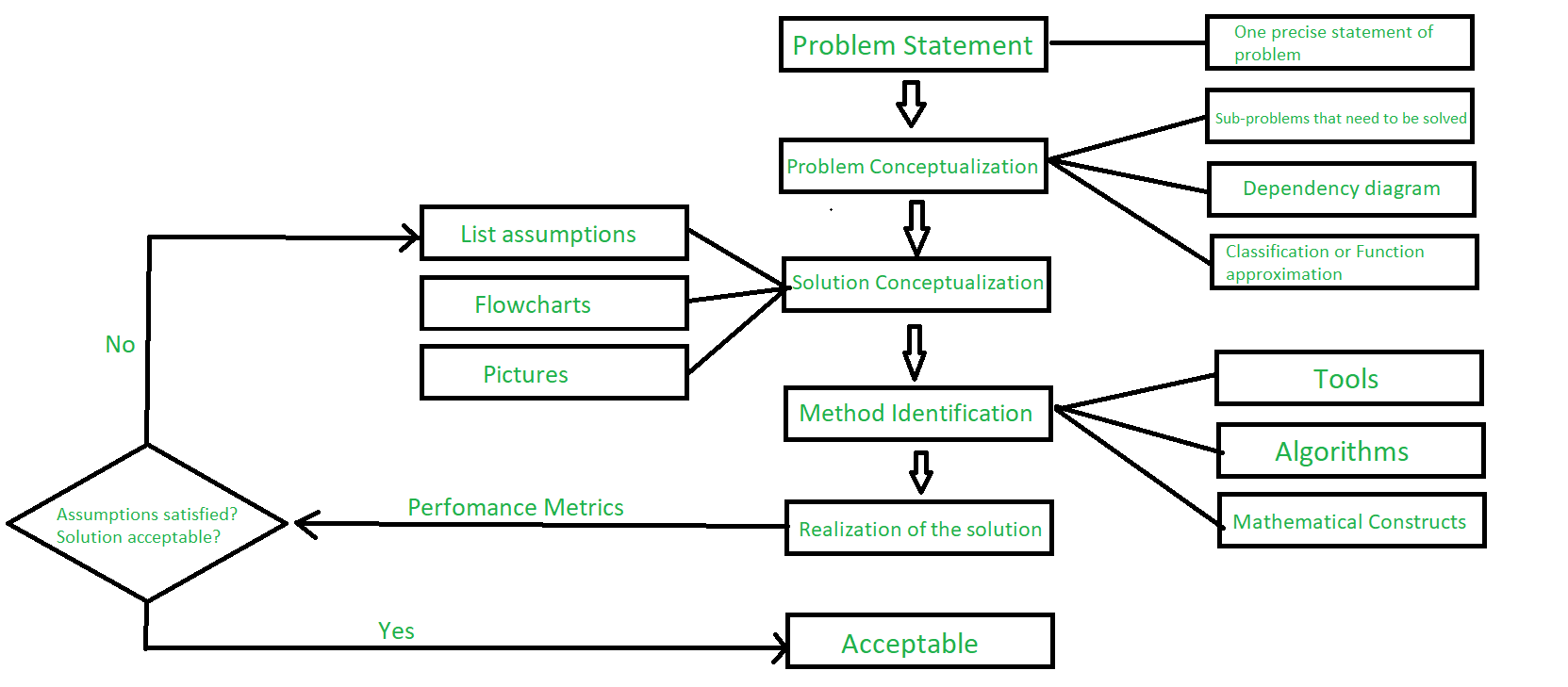# Conceptual Framework for Solving Data Analysis Problems

• Last Updated : 16 Jul, 2020

Data Science is an interdisciplinary field that focuses on extracting knowledge from data sets which are typically huge in amount. The field encompasses analysis, preparing data for analysis, and presenting findings to inform high-level decisions in an organization. As such, it incorporates skills from computer science, mathematics, statics, information visualization, graphic, and business.

Nowadays in many cases as far as the data analysis problems are concerned typically one starts with a very not well-defined problem, for example, one would say in a typical industrial scenario there is a feeling that there are lots of data that is around and everyone seems to suggest that, one should be able to use this kind of big data to derive some value to his/her organization. So, the question then is how does he/she do it?

So typically people start by saying there are lots of data, what he/she can do with this data? One might simply say he wants to improve performance or to minimize maintenance problems and so on. So, one could start talking about a class of problems which could be either performance-related or improving the operations doing things on time and so on. So, typically one starts with a loose set of words a vague definition of a problem, and the data that he/she has. Now the question is to then drive one’s thought process towards something that is codable, something that one can process the data to derive value to do any problem that he/she is solving and so on. While this is to some extent currently a little bit of unstructured process good data scientists come up with a solution flow that makes sense and that is relevant for the problem that needs to be solved.• Problem Statement: First of all one gets is the arrival of a problem having a lot of very diffuse problem statements. So step 1 is to convert this into one problem statement or set of problem statements as precise as possible and then to solve that problem one should do what is known as Problem Characterization/Problem Conceptualization.
• Problem Conceptualization: So, one breaks down this high-level problem statement into subproblems and kind of draws a flow process saying if he solves this subproblem then this result he is going to use in this subproblem and so on. So, one can think of this like a flowchart that he is drawing with these subproblems, and in general if possible he gets to a granularity level where he can identify the class of problem that the subproblems belong to either function approximation or classification problem. So one can identify these problems as either function approximation or classification problems.
• Solution Conceptualization: So, this is where one then looks at solution conceptualization. Again one has to make assumptions here. So, one could make assumptions about distributions about linearity and non-linearity the type of non-linearity, and so on. And here if one could draw a flowchart and have some pictures in his head then it becomes easier to solve this problem.
• Method Identification: Then once one conceptualize the solution then for each of these sub-models our submodules one has to identify a method and the identification of the method should be dictated by the assumptions that he has made before. One has to look at the assumptions and pick the right method for the solution and if it turns out that for the kinds of assumptions that he has made that he does not like any method that is out there then he tweaks the existing algorithms to a little bit and then find a method that is useful or that will work for his problem.
• Realization of the solution: Once one does this then he actualizes the solution in some software environment of choice and he then gets the solution and assesses whether the assumptions are good, whether the solution satisfies his requirements and if it does, he is done or if it does not he should go back and relook at his assumptions and then see how he changes or modifies his assumptions so that he gets a solution that he is comfortable with.
My Personal Notes arrow_drop_up